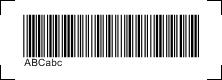# Barcode Code 39 / Code 39 Extended

Code 39 encodes numbers, capital letters (A-Z) and some special characters. By using combinations of two symbols the full ASCII character set can be encoded including special characters like currency symbols. If those combinations of symbols are used the code is referred to as Code 39 Extended. Our Barcode Generator will automatically generate the appropriate two symbol codes if it encounters a character from the extended set, e.g. a small cap.

Code 39 is mainly used for warehousing, in Germany and other countries it is also used for pharmaceutical products.

## Character Table Code 39 Extended

ASCII char, to the right the combination to encode. E.g., to encode a lowercase "a" into the code, one would have to actually encode "+A":

 NUL %U SUB \$Z 4 4 N N h +H SOH \$A ESC %A 5 5 O O i +I STX \$B FS %B 6 6 P P j +J ETX \$C GS %C 7 7 Q Q k +K EOT \$D RS %D 8 8 R R l +L ENQ \$E US %E 9 9 S S m +M ACK \$F SP Space : /Z T T n +N BEL \$G ! /A ; %F U U o +O BS \$H " /B < %G V V p +P HT \$I # /C = %H W W q +Q LF \$J \$ /D > %I X X r +R VT \$K % /E ? %J Y Y s +S FF \$L & /F § %V Z Z t +T CR \$M ' /G A A [ %K u +U SO \$N ( /H B B \ %L v +V SI \$O ) /I C C ] %M w +W DLE \$P * /J D D ^ %N x +X DC1 \$Q + /K E E _ %O y +Y DC2 \$R , /L F F ' %W z +Z DC3 \$S - - G G a +A { %P DC4 \$T . . H H b +B | %Q NAK \$U / /O I I c +C } %R SYN \$V 0 0 J J d +D ~ %S ETB \$W 1 1 K K e +E DEL %T CAN \$X 2 2 L L f +F DEL %X EM \$Y 3 3 M M g +G DEL %Z

## Checksum for Code 39

Code uses a modulo 43 checksum scheme. The characters are summed up, modulo divided by 43, the reminder is the check character.

## Sample Code 39 Extended## Create Code 39 Extended

Windows PC - Softmatic® BarcodePlus (Win, V4, Windows XP or higher)